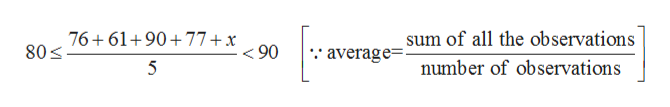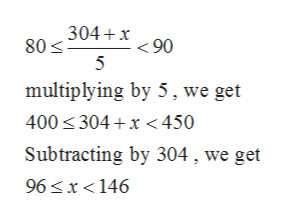A student must have an average​ (the mean) on five tests that is greater than or equal to​ 80% but less than​ 90% to receive a final grade of B.​ Devon's grades on the first four tests were 76​%, 61​%, 90​%, and 77​%. What range of grades on the fifth test would give him a B in the​ course? (Assume that​ 100% is the highest grade​ possible.)

Question

A student must have an average​ (the mean) on five tests that is greater than or equal to​ 80% but less than​ 90% to receive a final grade of B.​ Devon's grades on the first four tests were 76​%, 61​%, 90​%, and 77​%. What range of grades on the fifth test would give him a B in the​ course? (Assume that​ 100% is the highest grade​ possible.)

Step 1

Let x be the range of grade in fifth test.

Step 2

Then according to given conditions, we gethelp_outlineImage Transcriptionclose76+61 90 77 + x 80 sum of all the observations 90 average- number of observations 5 fullscreen
Step 3

Solving the above ineq...help_outlineImage Transcriptionclose304+x90 80 5 multiplying by 5, we get 400 304 x450 Subtracting by 304, we get 96 x<146 fullscreen

Want to see the full answer?

See Solution

Want to see this answer and more?

Our solutions are written by experts, many with advanced degrees, and available 24/7

See Solution
Tagged in

Equations and In-equations